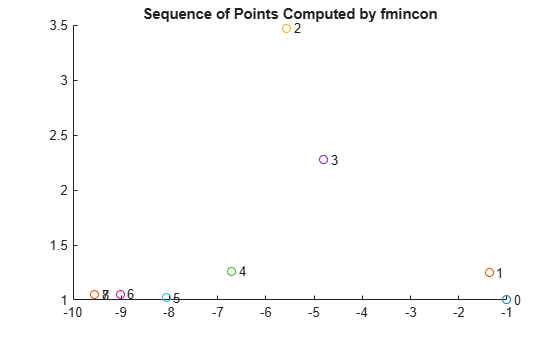# Output Functions for Optimization Toolbox™

### What Is an Output Function?

For some problems, you might want output from an optimization algorithm at each iteration. For example, you might want to find the sequence of points that the algorithm computes and plot those points. To do this, create an output function that the optimization function calls at each iteration. See Output Function and Plot Function Syntax for details and syntax.

This section shows the solver-based approach to output functions. For the problem-based approach, see Output Function for Problem-Based Optimization.

Generally, the solvers that can employ an output function are the ones that can take nonlinear functions as inputs. You can determine which solvers can have an output function by looking in the Options section of function reference pages.

### Example: Use an Output Function

This example shows how to use an output function to monitor the `fmincon` solution process for solving a constrained nonlinear optimization problem. The output function does the following at the end of each `fmincon` iteration:

• Plot the current point.

• Store the current point and its corresponding objective function value in a variable called `history`, and store the current search direction in a variable called `searchdir`. The search direction is a vector that points in the direction from the current point to the next one.

Additionally, to make the history available outside of the `fmincon` function, perform the optimization inside a nested function that calls `fmincon` and returns the output function variables. For more information about this method of passing information, see Passing Extra Parameters. The `runfmincon` function at the end of this example contains the nested function call.

### Objective and Constraint Functions

The problem is to minimize the function

`$f\left(x\right)=\mathrm{exp}\left({x}_{1}\right)\left(4{x}_{1}^{2}+2{x}_{2}^{2}+4{x}_{1}{x}_{2}+2{x}_{2}+1\right)$`

subject to the nonlinear inequality constraints

`$\begin{array}{l}{x}_{1}+{x}_{2}-{x}_{1}{x}_{2}\ge 3/2\\ {x}_{1}{x}_{2}\ge -10.\end{array}$`

The `objfun` function nested in `runfmincon` implements the objective function. The `confun` function nested in `runfmincon` implements the constraint function.

### Call Solver

To obtain the solution to the problem and see the history of the `fmincon` iterations, call the `runfmincon` function.

`[xsol,fval,history,searchdir] = runfmincon;`
``` Max Line search Directional First-order Iter F-count f(x) constraint steplength derivative optimality Procedure 0 3 1.8394 0.5 Infeasible start point 1 6 1.85127 -0.09197 1 0.109 0.778 ```
``` 2 9 0.300167 9.33 1 -0.117 0.313 Hessian modified twice ```
``` 3 12 0.529835 0.9209 1 0.12 0.232 ```
``` 4 16 0.186965 -1.517 0.5 -0.224 0.13 ```
``` 5 19 0.0729085 0.3313 1 -0.121 0.054 ```
``` 6 22 0.0353323 -0.03303 1 -0.0542 0.0271 ```
``` 7 25 0.0235566 0.003184 1 -0.0271 0.00587 ```
``` 8 28 0.0235504 9.035e-08 1 -0.0146 8.51e-07 ```
```Active inequalities (to within options.ConstraintTolerance = 1e-06): lower upper ineqlin ineqnonlin 1 2 ``````Local minimum found that satisfies the constraints. Optimization completed because the objective function is non-decreasing in feasible directions, to within the value of the optimality tolerance, and constraints are satisfied to within the value of the constraint tolerance. ```

The output function creates a plot of the points that `fmincon` evaluates. Each point is labeled by its iteration number. The optimal point occurs at the eighth iteration. The last two points in the sequence are so close that they overlap.

The output `history` is a structure that contains two fields.

`disp(history)`
``` x: [9x2 double] fval: [9x1 double] ```

The `fval` field in `history` contains the objective function values corresponding to the sequence of points `fmincon` computes.

`disp(history.fval)`
``` 1.8394 1.8513 0.3002 0.5298 0.1870 0.0729 0.0353 0.0236 0.0236 ```

These are the same values displayed in the iterative output in the column with header `f(x)`.

The `x` field of `history` contains the sequence of points that `fmincon` computes.

`disp(history.x)`
``` -1.0000 1.0000 -1.3679 1.2500 -5.5708 3.4699 -4.8000 2.2752 -6.7054 1.2618 -8.0679 1.0186 -9.0230 1.0532 -9.5471 1.0471 -9.5474 1.0474 ```

The second output argument, `searchdir`, contains the search directions for `fmincon` at each iteration. The search direction is a vector pointing from the point computed at the current iteration to the point computed at the next iteration.

`disp(searchdir)`
``` -0.3679 0.2500 -4.2029 2.2199 0.7708 -1.1947 -3.8108 -2.0268 -1.3625 -0.2432 -0.9552 0.0346 -0.5241 -0.0061 -0.0003 0.0003 ```

### Helper Functions

The following code creates the `runfmincon` function, containing the `outfun` output function, `objfun` objective function, and `confun` nonlinear constraint function as nested functions,

```function [xsol,fval,history,searchdir] = runfmincon % Set up shared variables with OUTFUN history.x = []; history.fval = []; searchdir = []; % call optimization x0 = [-1 1]; options = optimoptions(@fmincon,'OutputFcn',@outfun,... 'Display','iter','Algorithm','active-set'); [xsol,fval] = fmincon(@objfun,x0,[],[],[],[],[],[],@confun,options); function stop = outfun(x,optimValues,state) stop = false; switch state case 'init' hold on case 'iter' % Concatenate current point and objective function % value with history. x must be a row vector. history.fval = [history.fval; optimValues.fval]; history.x = [history.x; x]; % Concatenate current search direction with % searchdir. searchdir = [searchdir;... optimValues.searchdirection']; plot(x(1),x(2),'o'); % Label points with iteration number and add title. % Add .15 to x(1) to separate label from plotted 'o' text(x(1)+.15,x(2),... num2str(optimValues.iteration)); title('Sequence of Points Computed by fmincon'); case 'done' hold off otherwise end end function f = objfun(x) f = exp(x(1))*(4*x(1)^2 + 2*x(2)^2 + 4*x(1)*x(2) +... 2*x(2) + 1); end function [c, ceq] = confun(x) % Nonlinear inequality constraints c = [1.5 + x(1)*x(2) - x(1) - x(2); -x(1)*x(2) - 10]; % Nonlinear equality constraints ceq = []; end end```